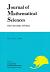## Vol. 8 (2001) No. 04Journal of Mathematical Sciences The University of Tokyo

1. Liu, Deming
On the Holonomic Deformation of Linear Differential Equations of the $A_g$ Type
Vol. 8 (2001), No. 4, Page 559--594.

2. Sugiki, Yuichi
Division Theorems in Higher-codimensional Boundary Value Problems for $\cal E$-modules
Vol. 8 (2001), No. 4, Page 595--608.

3. Tsuzuki, Masao
Real Shintani Functions on $U(n,1)$
Vol. 8 (2001), No. 4, Page 609--688.
Real Shintani Functions on $U(n,1)$ II, Computation of Zeta Integrals
Algebraic Formulae for the $q$-inverse in a Free Group